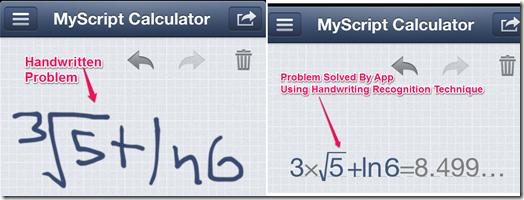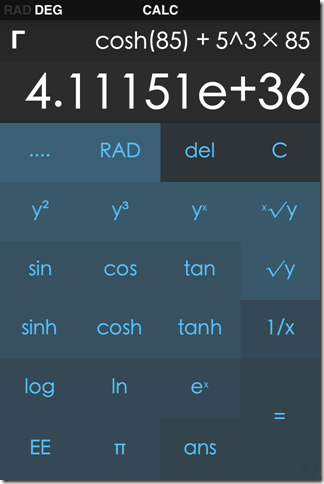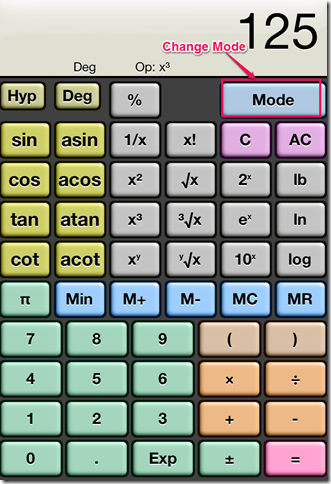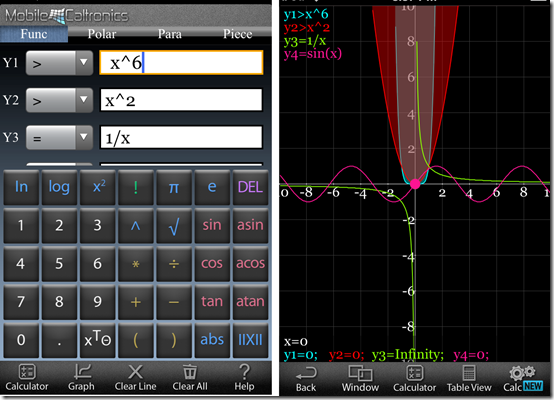Editor Ratings:
User Ratings:
[Total: 0 Average: 0]

Here is a list of 5 free scientific calculator apps for iPhone. You can use these apps to solve complex mathematical problems apart from the traditional mathematical problems.

These calculators let you solve problems like trigonometry, exponential, factorials etc. which are not possible with the use of native calculator app for iPhone. If you are a mathematics/engineering student, any professional or a tutor, then these apps can prove to be very useful for you on daily basis.

## 1. MyScript Calculator:The first scientific calculator app for iPhone is MyScript Calculator. You can perform both simple as well as scientific calculations using this calculator app. The most interesting and cool feature of the app is that it works on handwriting recognition technique. It means that there is no keyboard for typing the problems. You just need to use your handwriting to input the problems and the app recognizes your handwriting to solve the problem. This calculator supports ratio, powers, logs, trigonometric ratios, inverse trigonometry, roots, and constants.

## 2. Calculator+:Calculator+ is the second app in the list. This scientific calculator app for iPhone lets you solve problems like factorials, cube root, square root, exponential, natural log, log with base 10, log with base 2, trigonometric, etc. You can also customize the app with two free themes (White and Black) while about 20 themes are available with paid version. The free version lets you check the preview of the themes but you can’t apply them. Similarly, this app also has in-built handwriting calculator, polynomial calculator, linear calculator, graphing calculator, and currency calculator but all these are available only with paid version of the app.

## 3. CALC – A Scientific Calculator:The third app in the list is CALC – A Scientific Calculator. The unique thing about this calculator is that it performs all the calculations in real-time. It means if you are calculating a problem involving multiple mathematical operations then, it displays the result simultaneously. You don’t have to wait till the end for pressing the equal sign to get the results. In the settings menu, you can select the number of decimal places, clock while using CALC, and language. It also has a Wiki section where you can check the meaning of abbreviations used on the keys of the calculator.

## 4. Kalkulilo (Scientific Calculator):Kalkulilo is a very powerful and multi-purpose scientific calculator app for iPhone. This app comes with 6 in-built modes: Simple Calculator, Scientific Calculator, Bit/Integer, Statistics Calculator, Trigonometry Calculator, and Base/Radix Calculator. You can switch between these calculators easily by pressing the MODE key. The app lets you set the exponents setting for the Scientific/Statistics Mode and number of bits for Bit/Integer mode. Besides this, you can also customize the calculator using different colors and key styles.

## 5. iMathPac:The last app in the list is iMathPac. You can use this app as scientific calculator as well as to view the graphical representations of equations. The app has support for four types of equations: Functions, Polar, Para, and Piece. The first one is the free one while the rest are the paid ones. Apart from this, the app has support for all the scientific functions like sin, cos, tan, permutations, combinations, cube root, square root, power, factorial, exponential etc.

These were the 5 free scientific calculator apps for iPhone. Check them out so that you don’t have to spend long time in solving the scientific problems. Do share the name of the app which you liked the most.

 Editor Ratings: User Ratings:[Total: 0 Average: 0] Works With: iPhone, iPad, and iPod Touch Free/Paid: Free